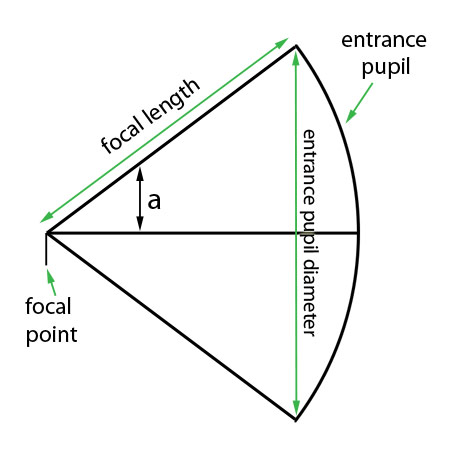# Numerical Aperture

back

The numerical aperture is the same concept as f/number but on the front of the lens. Whereas the f/number determines the image resolution, the numerical aperture determines the object resolution. Numerical aperture is the angle that the aperture makes with the front focal point.

The basic form of numerical aperture is determined by two measurements - the size of the aperture and the distance that the aperture is from thefront focal point of the lens. A larger aperture that is closer to the focal point will have a larger numerical aperture (NA) and will result in higher potential object resolution. The NA is closely associated with the f/number of the lens.

NA = 1/(2*f-num) (where f-num = focal length/entrance pupil diameter)

and vice-versa: f-num = 1/(2*NA)

Technically, the numerical aperture is the sine of the half angle that the aperture makes with the front focal point. I have seen various sources suggest that for small NA's the tangent of the angle is a close approximation of the sine. While that assertion is true, it is superfluous. Why use an approximate tangent when the sine is correct? Maybe somebody can enlighten me.

At first glance, it would appear that the NA is equal to the tangent of the angle as the NA is equal to half of the entrance pupil diameter divided by the distance from the entrance pupil to the focal point. The first time I looked at a typical diagram, I thought the same: Why sine and not tangent?The answer lies in the fact that the entrance pupil of a well-corrected photographic lens is not just a circle, it is a section from a sphere with a diameter f (focal length)centered at the focal point of the lens (based on a quote from Rudolph Kingslake - a well respected optical engineer so I'll go with that).

That means that the hypoteneuse of my triangle is equal to the focal length. The vertical line down to the baseline of the triangle from the edge of the entrance pupil (En/2 in length). That means that the NA represents the sine of the angle and not the tangent.NA = sin(angle a) = (En/2)/focal length (where En is the entrance pupil diameter)

Like f-number and effective aperture on the backside of the lens, there is NA and effective NA on the front. It is likewise affected by the magnification and the pupillary magnification. We will go into that concept in the next posting.